# My Notes: Memory (CS50)

### 4/21/2020

We will look at different base systems. Hexadecimal (base 16), 0-F.

Binary is powers of 2, decimal is powers of 10, hexadecimal is powers of 16 (so you can count more/higher).

If you've worked with CSS or styling, hexadecimal values, RGB.

`#FF0000` FF is a lot of Red, no green, no blue

To avoid any confusion with decimal system, it was decided to prefix any hexadecimal with a 0x.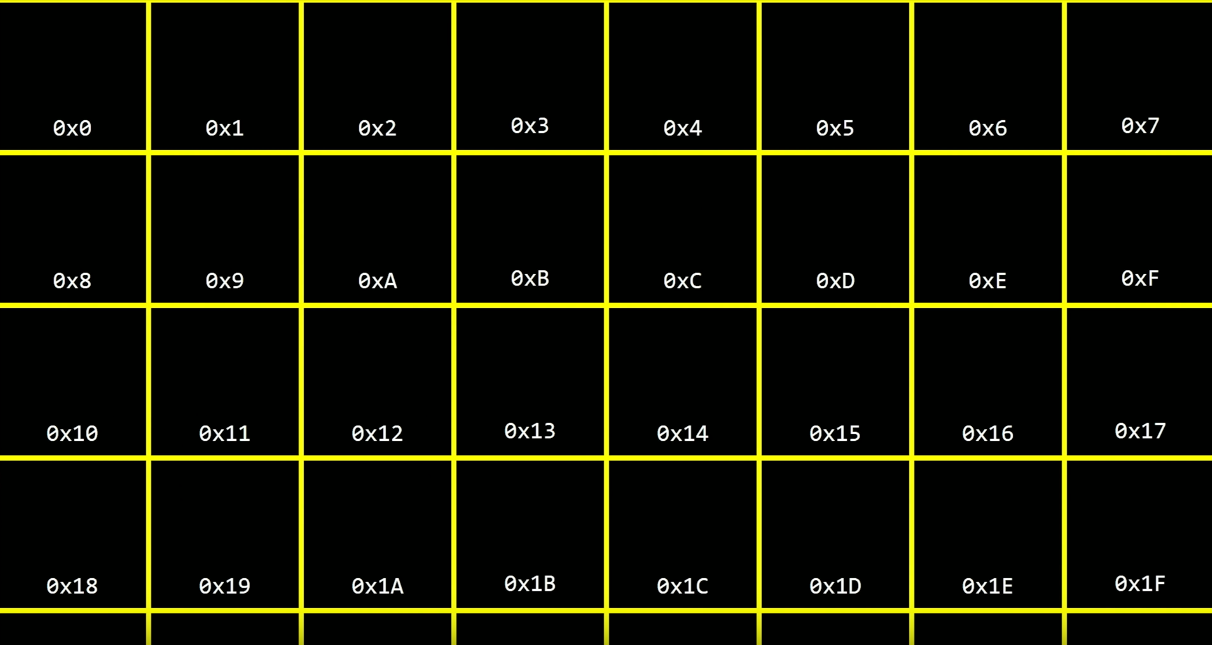### Locations in Memory

Variables are very common in programming. In a program, we can just set a variable to a value and print it to console, as seen below.

``````#include <stdio.h>

int main(void)
{
int n = 50;
printf("%i\n", n);
}
``````

This will just print out `50`.

If we want to find out where the `n` variable is stored in memory, we can change the program to as follows.

``````#include <stdio.h>

int main(void)
{
int n = 50;
printf("%p\n", %n);
}
``````

We change the placeholder from `%i` to `%p` and add an `&` in the `printf()` function. It points to the value in memory.

Another operator which is useful is *. It is the opposite of &.

`&` is what is the address (in memory)

`*` is go to the address (in memory)

We can also take n's place in memory and store it in a variable. We must prefix the variable name with an `*` so we know its a pointer.

``````int n = 50;
int *p = &n;
printf("%p\n", p);
``````

Locations in memory are a little low level, we are just concerned that the memory address points to the correct variable.

### Strings & Memory

``````string s = "EMMA";
``````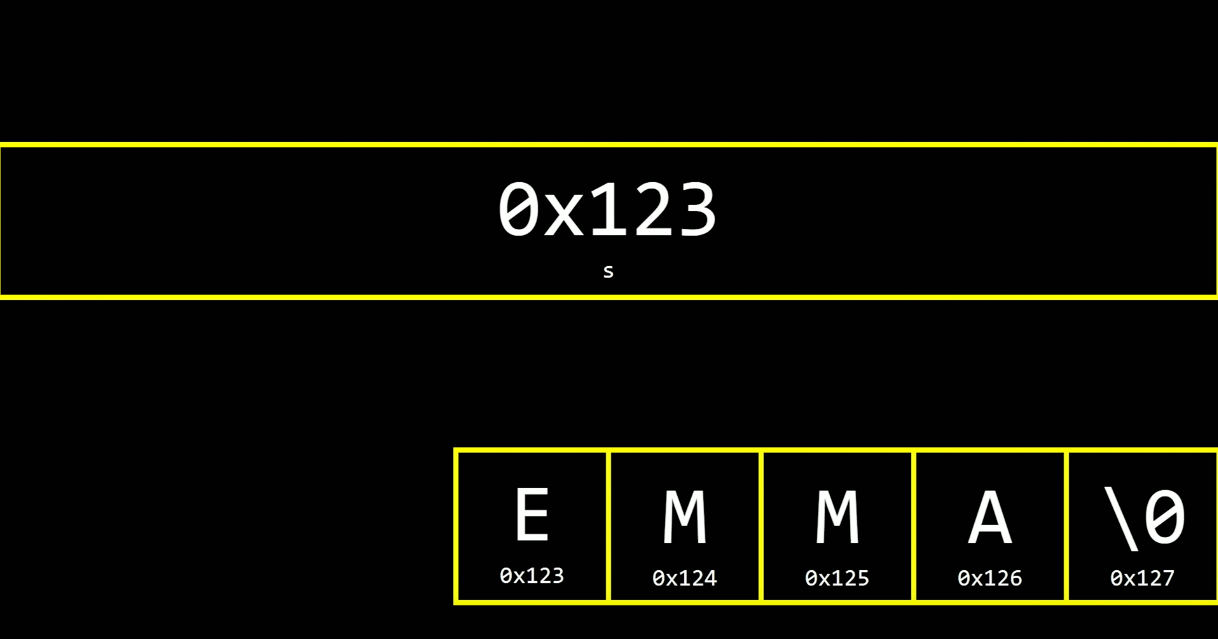s is a pointer to Emma's name in memory, specifically at the beginning of her name.

How does the computer know where the name ends if it just knows the beginning? The null `\n` terminator. The computer is smart enough, if it knows the starting of the string, can figure out where it ends.

Is string really a data type then?

``````char *s = "EMMA";
``````

The above code would point to the first character in the string, and the computer can read the whole string and see once it reaches the null terminator, the string has ended.

The below code is from the cs50 library. Creates a type called `string`, which is a pointer to the `char`.

`typedef char *string;`

A string is a char star. A string is a variable that contains the address of a character.

``````#include <cs50.h>
#include <stdio.h>

int main(void)
{
string s = "EMMA";
printf("%s\n", s);
}
``````

With a few changes, this will replicate same output below. We don't need cs50 library, and we can just use char *s as there is no typdef string anymore.

``````#include <stdio.h>

int main(void)
{
char *s = "EMMA";
printf("%s\n", s);
}
``````

We can print out each character in "EMMA" to show they're stored next to each other.

### Comparing Strings

``````#include <cs50.h>
#include <stdio.h>

int main (void)
{
string c = get_string("c: ");
string d = get_string("d: ");

if (c == d)
{
printf("Same\n");
}
else
{
printf("Different\n");
}

}
``````

Even with the same exact strings, we always get "Different". Why?

The strings are stored in different locations in memory. The variables that hold strings actually just hold the memory location address that point to the characters stored.

### `char *s` is `string`

From earlier, char *s points to the location of the first character location.

If we set a variable to a string and try to copy that variable into another variable, we run into issues since its sharing the same memory space. There is a work around to this, lets explore below.

``````#include <cs50.h>
#include <ctype.h>
#include <stdio.h>

int main (void)
{
char *s = get_string("s: ");
char *t = s;

t = toupper(t);

printf("%s\n", s);
printf("%s\n", t);
}
``````

If you enter "bob" for s, s gets copied to t. They are both in the same memory space. You uppercase Bob in the variable t. When you print, both give back "Bob".

The solution is to allocate memory to the new variable, then we can copy over the string.

``````#include <cs50.h>
#include <ctype.h>
#include <stdio.h>
#include <string.h>
#include <stdlib.h> // CS50 IDE mentioned I need this

int main (void)
{
char *s = get_string("s: ");
char *t = malloc(strlen(s) + 1); // memory allocation

strcpy(t, s); // function instead of for loop

t = toupper(t);

printf("%s\n", s);
printf("%s\n", t);
}
``````

So we take a user input for `s`. The t variable is dynamic as it will allocate the length of `s` plus one space for the null terminator.

malloc has a return value. malloc returns to the first byte (pointer). There is a bug in our problem. We allocate memory but we're not freeing it.

There is a command, `free`. It frees up memory to prevent all the memory being taken up. How to find where theres memory being taken up. There is another debugging method, `valgrind`.

Valgrind is a tool, built in to CS50 IDE, to help identify where there are memory leaks.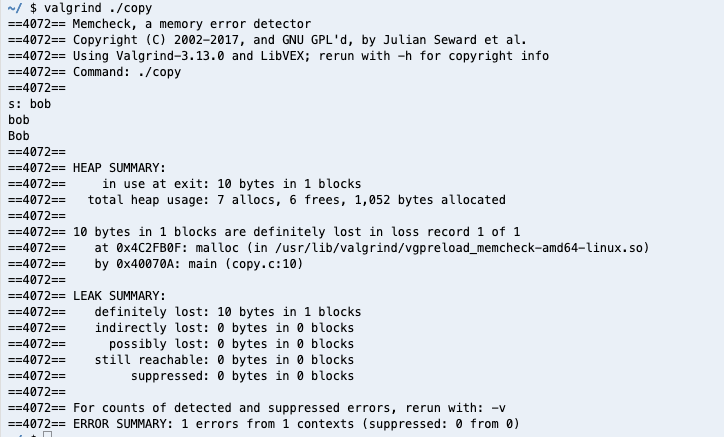To fix this issue, we can call `free(t)` in our program to free up memory allocation for `t`.

Buffer Overflow -- Regarding memory/array of memory, if you go over one value over, its an overflow.

### Swapping Values

If we have two values, and want to swap them, how would we go about it? Maybe if we have a and b, we can make a third variable, to hold one temporarily. Lets try --

``````#include <stdio.h>

void swap(int a, int b); // Prototype of swap function

int main(void)
{
int x = 1;
int y = 2;

printf("x is %i, y is %i\n", x, y);
swap(x, y);
printf("x is %i, y is %i\n", x, y);
}

void swap(int a, int b)
{
int tmp = a;
a = b;
b = tmp;
}
``````

When you pass inputs into functions, you're passing copies of the inputs. `swap()` works, but it doesn't swap x and y since they are just copies.

C uses your computer's memory in a methodic way. On the top, all the machine code goes on top. Below machine code, globals will go under. Heap is under globals, its a big chunk of memory, where you can allocate memory. If you call malloc, it is taken from the heap. Anytime you call a function, the function's local variables, go at the bottom of the memory. It is called the stack.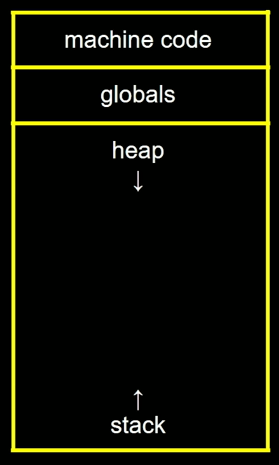With stacks, its like a dining hall tray system...once the function is ran, its taken off the stack.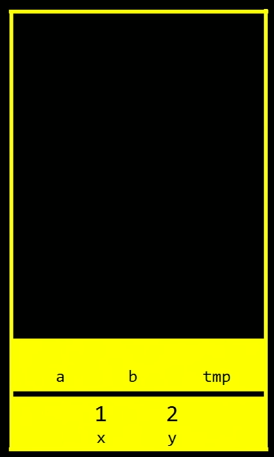Swap did not work because it was passed copies of x, y. How to solve this?

Pass by reference. References are like pointers from earlier.

Lets tell the function the addresses instead of a copy of the values.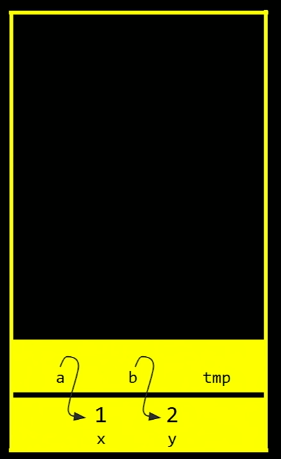Here is the swap() code from earlier, re-written. We are taking addresses of x, y.

``````#include <stdio.h>

void swap(int *a, int *b); // Prototype of swap function

int main(void)
{
int x = 1;
int y = 2;

printf("x is %i, y is %i\n", x, y);
swap(&x, &y);
printf("x is %i, y is %i\n", x, y);
}

void swap(int *a, int *b)
{
int tmp = *a;
*a = *b;
*b = tmp;
}
``````

We provide addresses instead of copies of values. The program is now working as intended. Using `*a` and `*b` is instructing the program to use pointers instead of value copies.

If you keep using malloc() and calling functions, the stack and the heap will keep on growing. We will face overflow.

-Heap Overflow (excessive memory allocation)

-Stack Overflow (excessive function calling)

The built in functions in the CS50 library. They deal with memory allocation, working with pointers, etc.

• get_char()
• get_string()
• get_int()

Without something like, get_int(), our program would look like this (w/o CS50 library)

``````#include <stdio.h>

int main(void)
{
int x;
printf("x: ");
scanf("%i", &x);
printf("x: %i\n", x);
}
``````

If we try making a program with strings (instead of get_string), we run into some problems --

``````#include <stdio.h>

int main(void)
{
char *s;    //char s would fix this;
printf("s: ");
scanf("%s", s);
printf("s: %s\n", s);
}
``````

s is not intialized. We need to make some space. We can intialize s an array with a fixed size just to try. Arrays are blocks within the memory so they work well with storing strings since strings are back to back characters.

### Phonebook

We're going to store a file! We're going to add name and phone numbers to a file, and keep updating it.

``````#include <cs50.h>
#include <stdio.h>
#include <string.h>

int main(void)
{
FILE *file = fopen("phonebook.csv", "a");
// a is for append (there is read and write as well)

char *name = get_string("Name: ");
char *number = get_string("Number: ");

fprintf(file, "%s,%s\n", name, number); // print to a file

fclose(file);
}
``````

If you run this, it will create a .csv file and will keep updating.